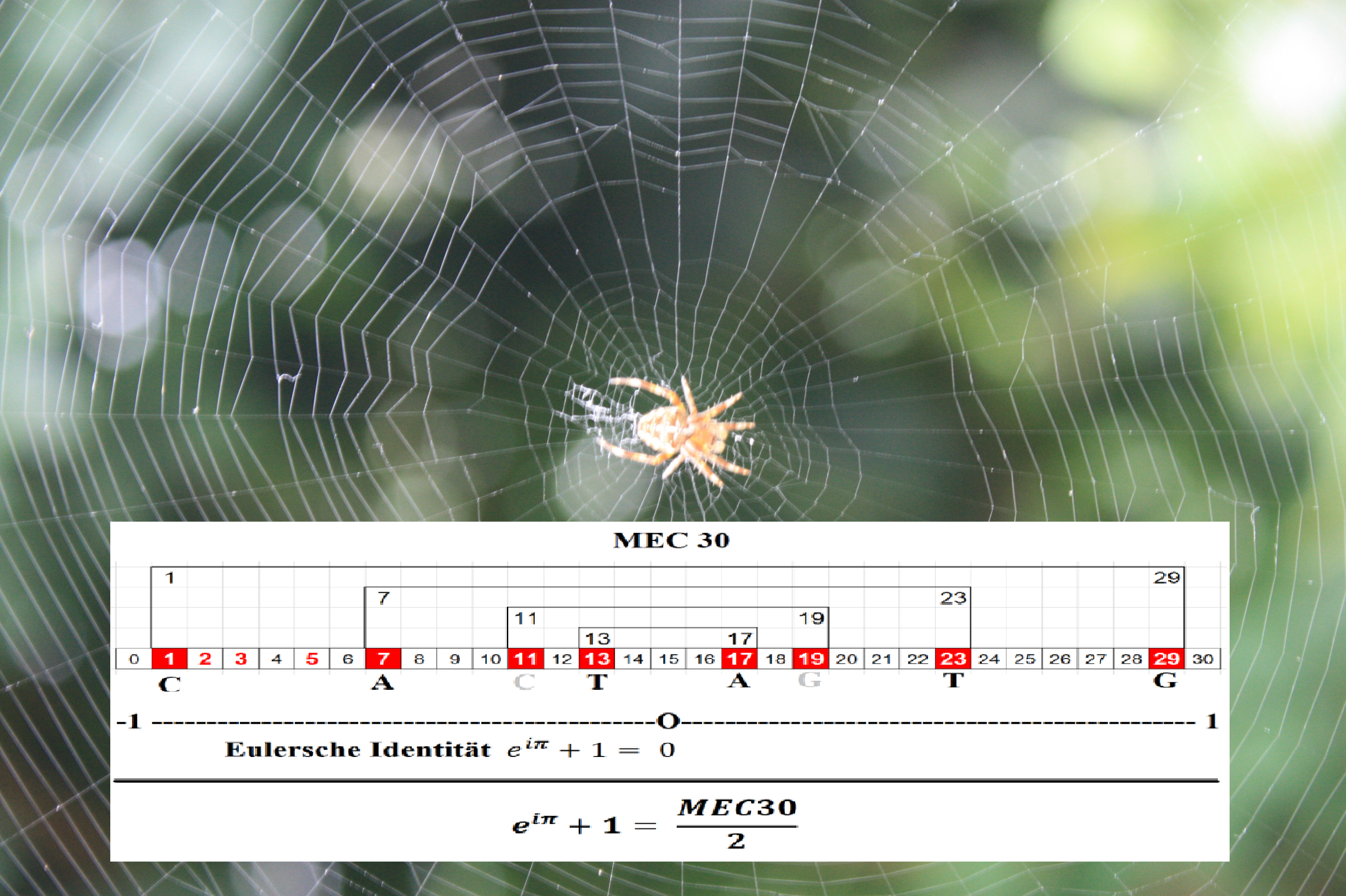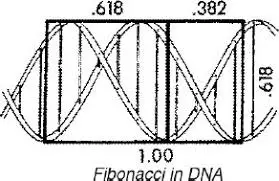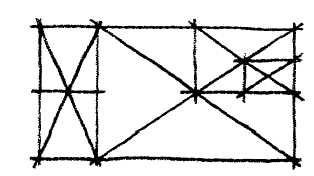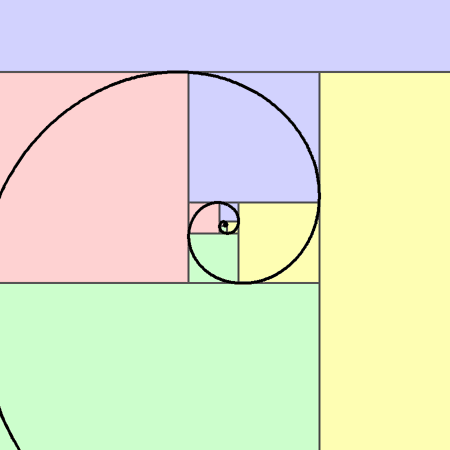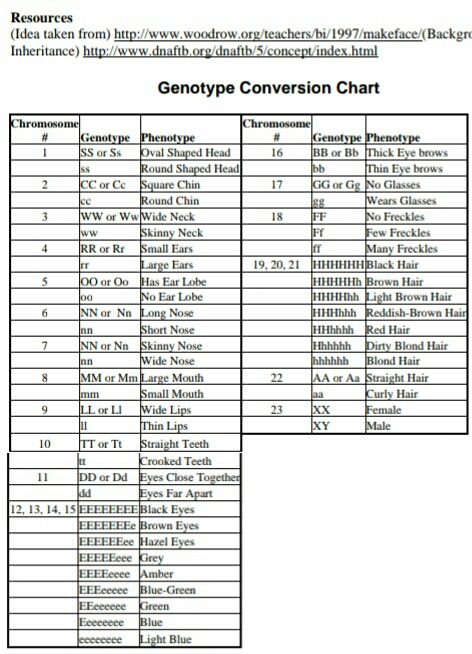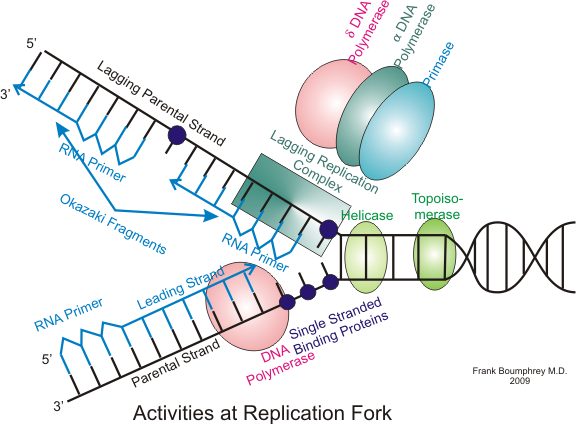96 is the smallest integer n for which π(n) = n/4.

π(96) = π(4x24) = 24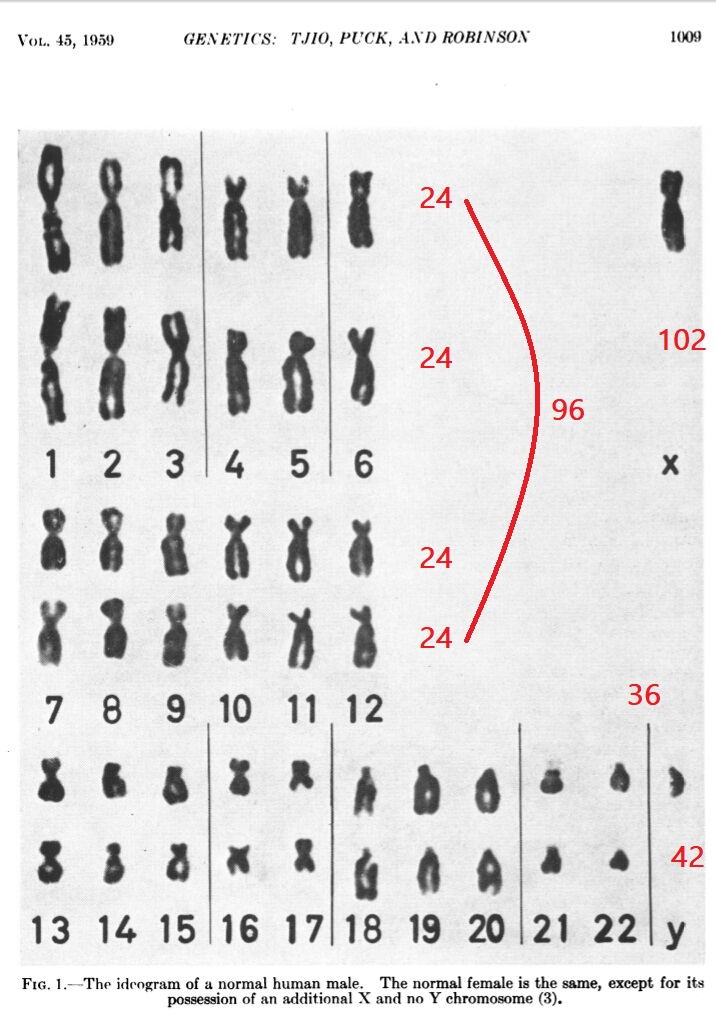139 - (25-18-18-25) = (114-18) - 18 - 25 = (96-18) - 25 = 78 - 25 = 53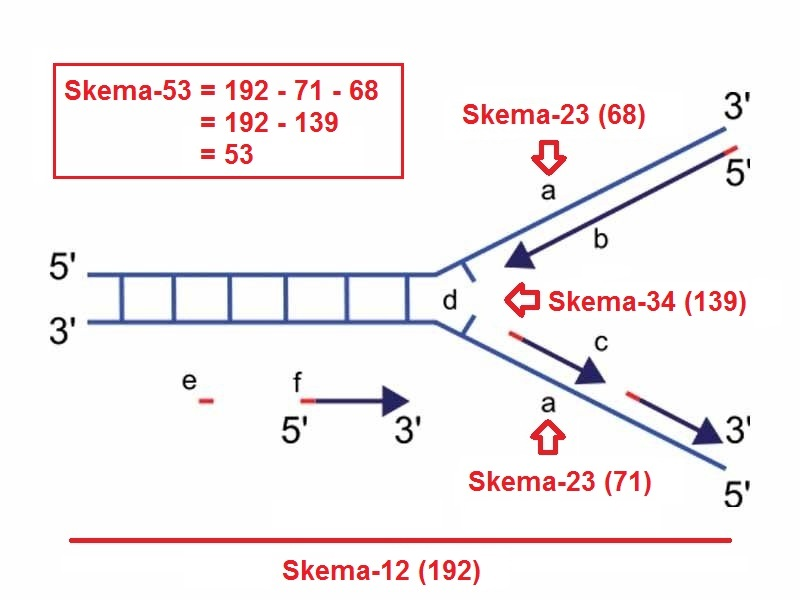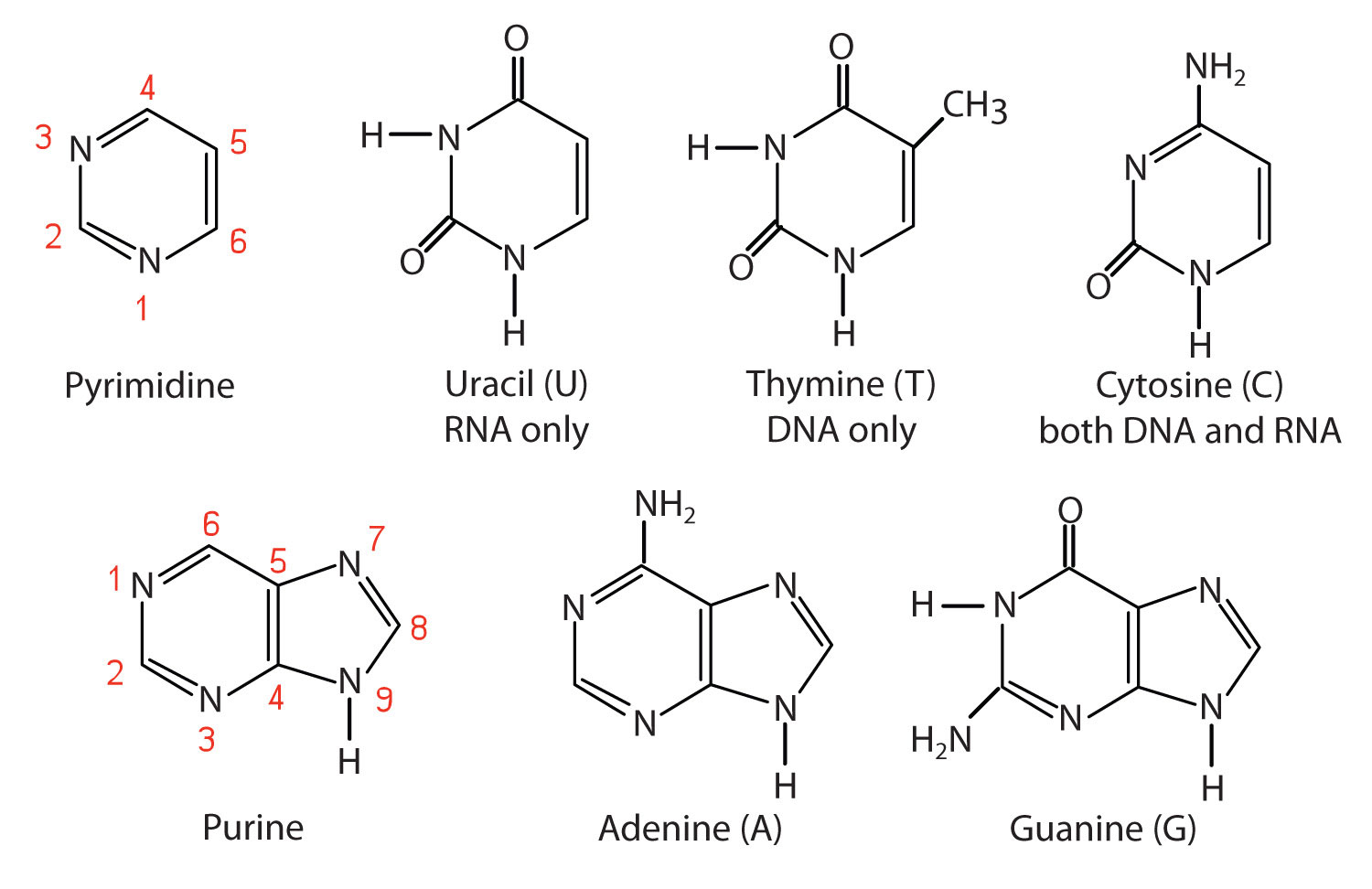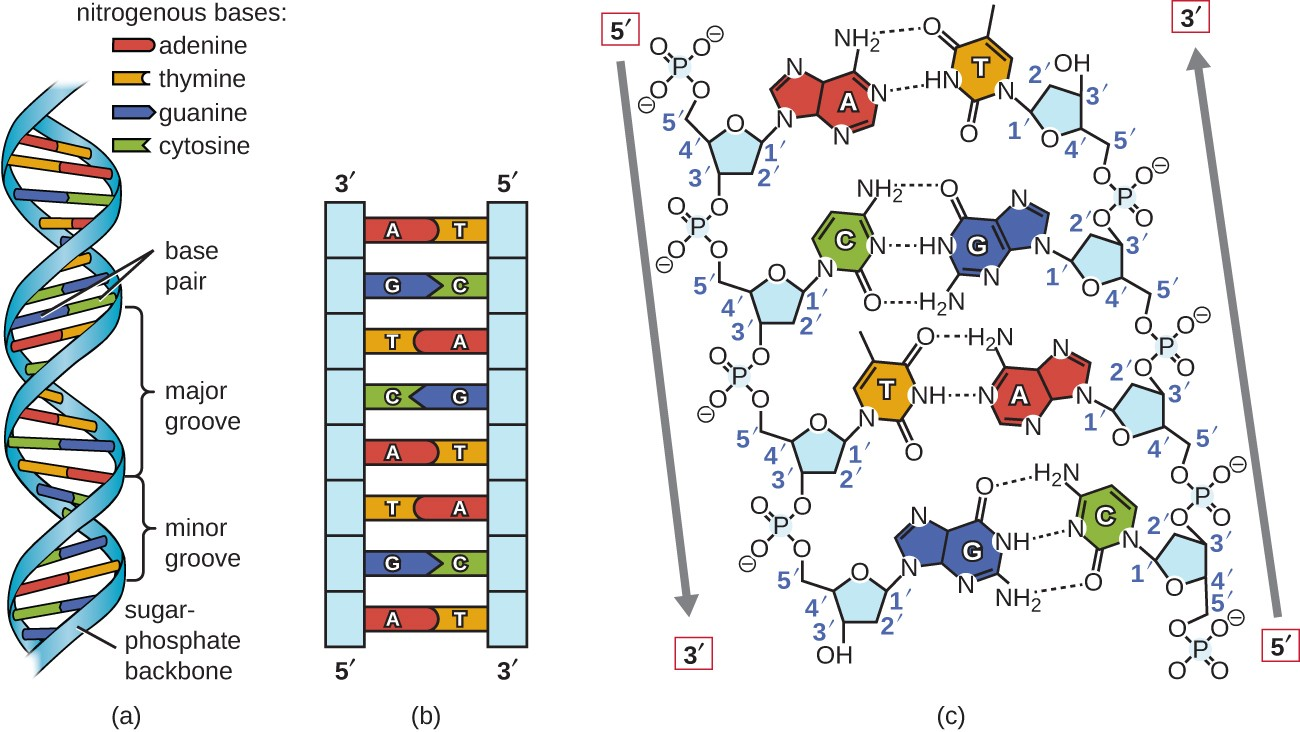See that from 1 to 89 it consist of seven (7) steps which is originated by 1,15,35,28 before it goes bilateral.The distribution of these scale has a bilateral symmetrical relationship along with the peculiarities of each numbers within the format (18,30,36) of prime hexagon so that it can represent the pattern of true-prime-pairs systemically.

(5 + 7 + 11 + 13) + (17 + 19) = 36 + 36 = 72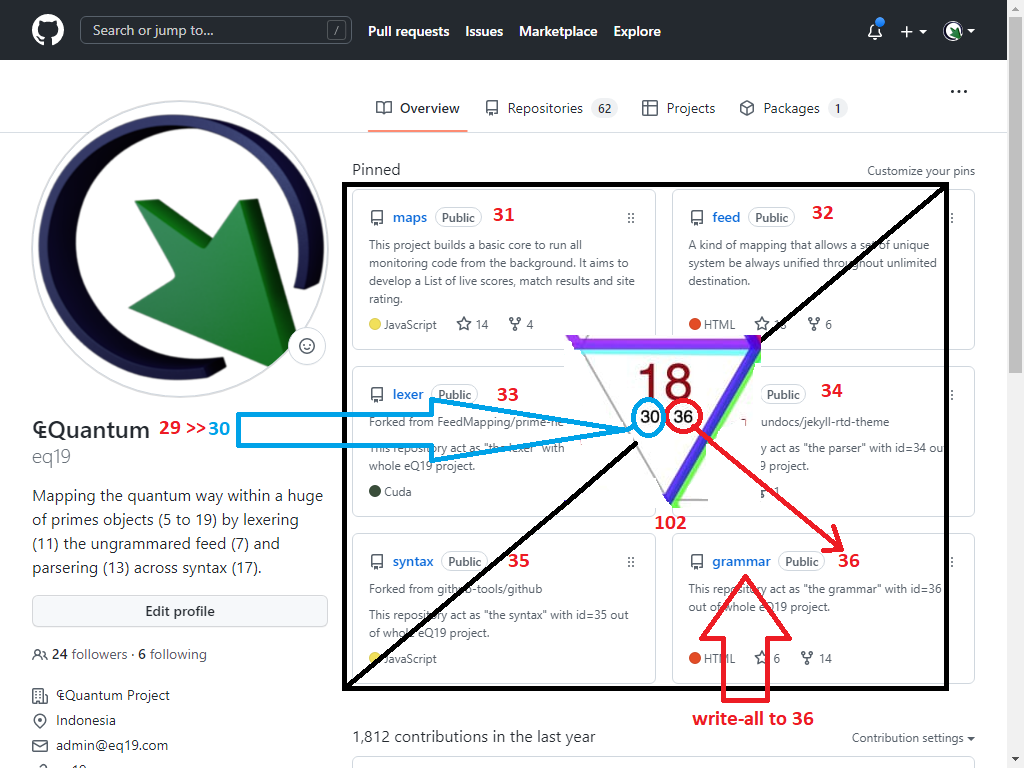This 29 turns the finiteness position of 15 as the middle zero axis of the MEC30. So the next steps will start exactly with the same story as we have explained from the beginning.

MEC 30 claims to "illustrate and convey the connections between quantum mechanics, gravitation and mathematics in a new way" via the elementary level of numbers. It starts with a theory about the structure of light, which is then transferred to various areas of the natural sciences.